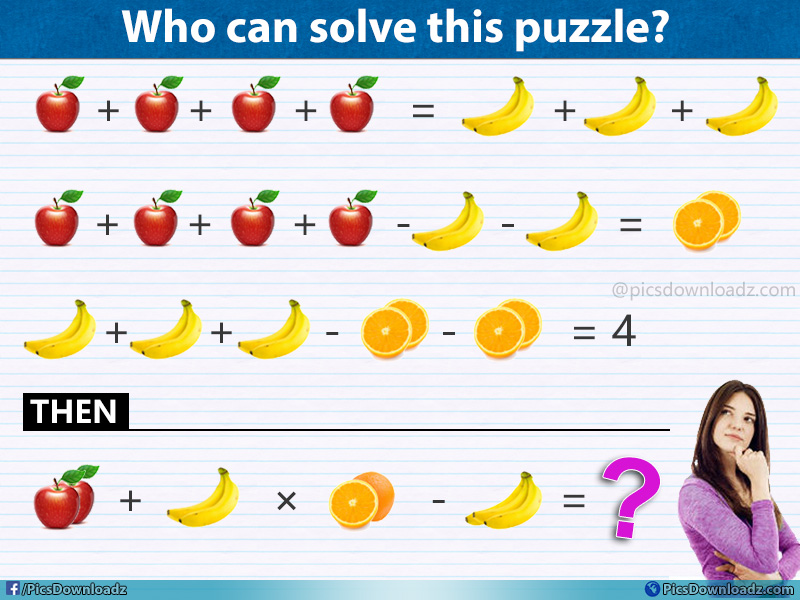This post may contain affiliate links. This means if you click on the link and purchase the item, We will receive an affiliate commission at no extra cost to you. See Our Affiliate Policy for more info.

Apple + Banana x Orange + Banana = ??  – Viral Fruits Math Puzzle

0
3702

Puzzle for geniuses! 90% will fail to solve this interesting fruit math puzzle. Viral apple banana orange math puzzle with the answer!

Here is another genius fruit math puzzle for you. This puzzle is divided into four equation, solve the first three equations and find the values of each fruit of the puzzle and then put the value in the last equation and find the correct answer. Let see how many of you can solve this fruit puzzle. The comment section is all yours, best of luck!

Apple Banana Orange – Viral Fruits Math Puzzle Image:Share your answer in the comment section below before you confirm it or keep scrolling for the answer and solution.

.

A

N

S

W

E

R

.

Let us consider:

Apple = A

Orange = O

Banana = B

Equation 1:

⇒ A + A + A + A = B + B + B

In this equation, we can’t find out the value of Apple and Banana. Let’s figure out the other equations

Equation 2:

⇒ A + A + A + A – B – B = Orange

We can rewrite the equation like;

⇒ A + A + A + A  = B + B + Orange

Now if we compare this equation with the first one, we get;

Banana = Orange

Equation 3:

⇒ B + B + B – O – O = 4

Therefore; Banana = 4 = Orange (O)

Now the 1st Equation:

4 Apple = 3 Banana

4 Apple = 3 x 4

Apple = 12/4 = 3

Apple = 3

Equation 4:

⇒ 2 Apple + Banana x Orange – Banana =??

In 4th Equation, if you noticed there are three banana in the equation, also if you compare the Oranges from first two equation with the last one you will find the difference. In first three equation there are 2 half oranges but the last equation it’s One + Half

Now rewrite the 4th equation:

⇒ 2 Apple + B (2 Bananas) x Orange (One + Half) – B (3 Bananas) =??

Fruit Values as follow:

One Apple = 3

2 Banana = 4   (From 3rd Equation), therefore One Banana = 4/2, One Banana = 2

Orange (Half + Half) = 4   (From 3rd Equation, Banana = Orange)

so we get, Half Orange = 2 and Full Orange = 4

Now, Final Equation

⇒ (2 Apples) + (2 Bananas) x (Full + Half Orange) – (3 Bananas) =??

⇒ Apple (3 + 3) + Banana (2 + 2) x Orange (4 + 2) – Banana (2 + 2 + 2) =??

⇒ 6 + 4 x 6 – 6 =?? (Now do multiplication first)

⇒ 6 + (4 x 6) – 6 =??

⇒ 6 + 24 – 6 =??

Hope, you found this puzzle interesting. 😉

LIKE | SHARE | PIN IT

Share this genius puzzle in your group let see how many of them can solve this puzzle. Like our fb page and join our puzzle group for more interesting puzzle image. Enjoy Take Care!

Like our Page: